# OECD Nutrient balance data analysis 8 - F-Test and Heteroskedasticity-Robust Inference in RPhoto by S. Tsuchiya on Unsplash

This post is following above post.

In the previous post, I did multiple regression, s_ni_kg ~ s_po_kg + s_ni_to.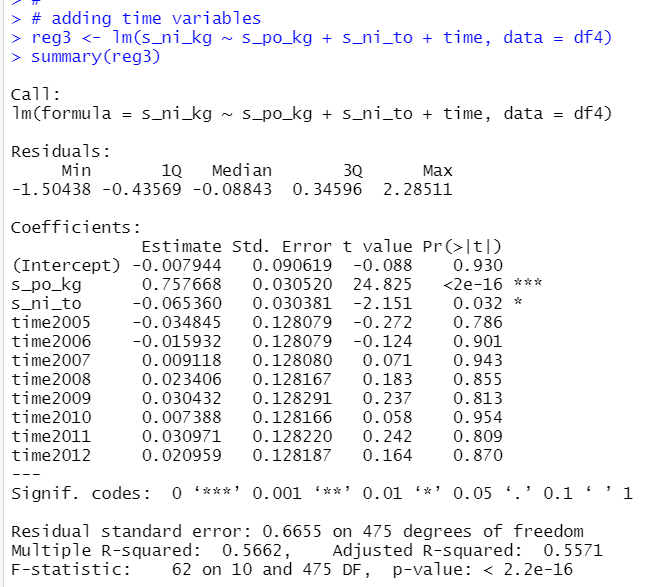All time variables are not statistically significant by individual variable base.
Let's check if all time variables are jointly significant or not.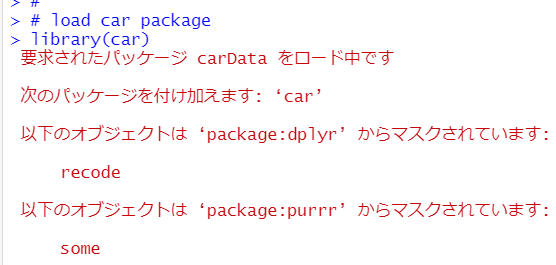Next, I use linerHypothesis() function.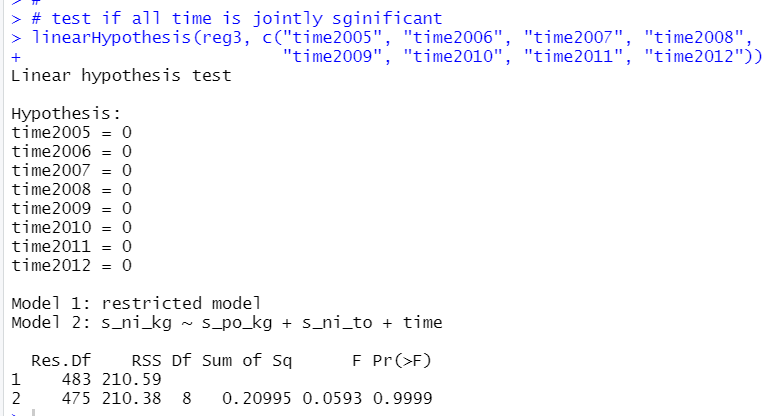p-value is 0.9999, so Null Hypothesis: All time variables are 0, cannot be rejected.
It means time is jointly insignificant.

Then, let's check if there is heteroskedasticity or not.

Fisrstly, manual method.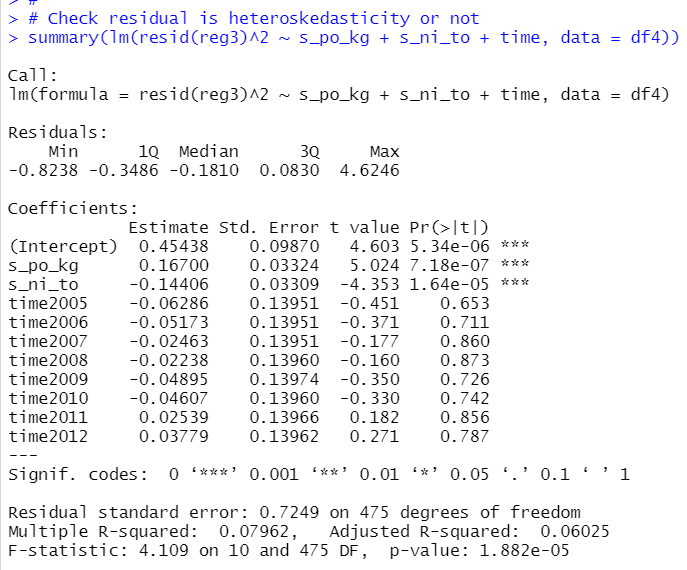p-value is very less than 0.05. So, I see there is heteroskedasticity in reg3.

Next, let's use lmtest packages's bptest().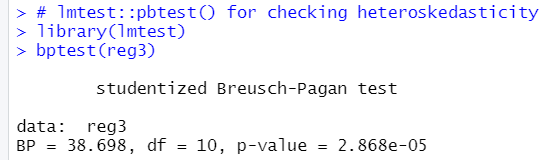p-vallue is less than 0.05. So, there is heteroskedasticity.

If there is heteroskedasticity, er cannot use normoal inference,

so we need heteroskedasticity robust inference.

I use lmtest::coeftest() function and car::hccm().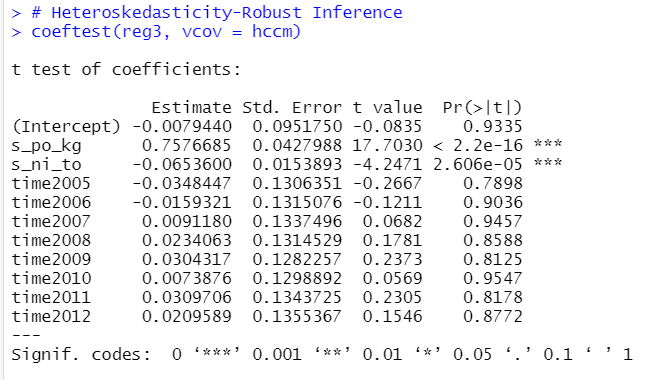We see s_po_kg and s_ni_to are still significant variables.
That's it. Thank you!

To read from the first post,